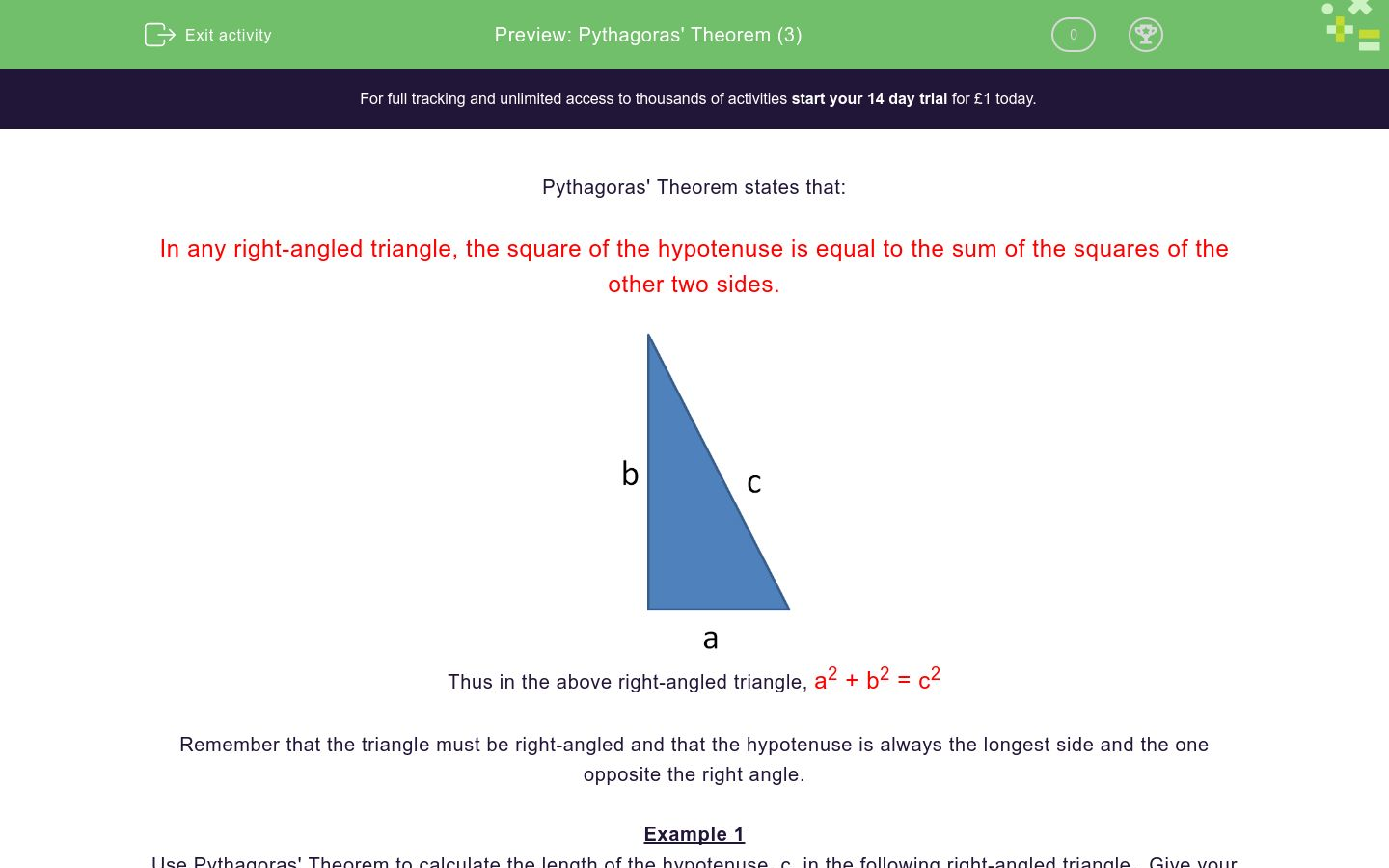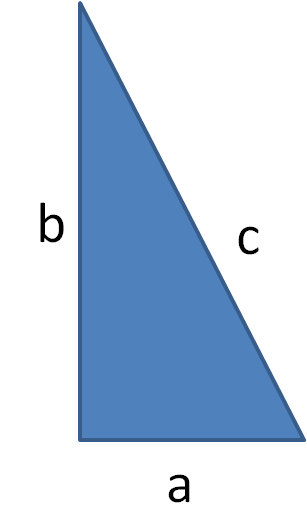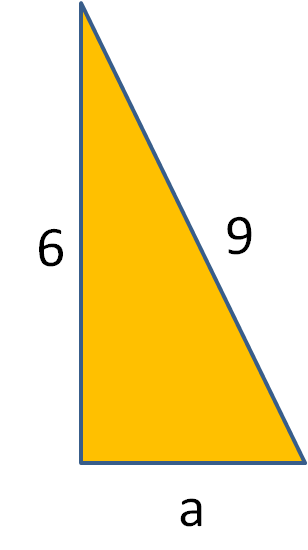# Pythagoras' Theorem (3)

In this worksheet, students find the length of the missing side of right-angled triangles using Pythagoras' Theorem.Key stage:  KS 3

Curriculum topic:   Geometry and Measures

Curriculum subtopic:   Solve Right-Angled Triangle Problems

Difficulty level:### QUESTION 1 of 10

Pythagoras' Theorem states that:

In any right-angled triangle, the square of the hypotenuse is equal to the sum of the squares of the other two sides.Thus in the above right-angled triangle, a2 + b2 = c2

Remember that the triangle must be right-angled and that the hypotenuse is always the longest side and the one opposite the right angle.

Example 1

Use Pythagoras' Theorem to calculate the length of the hypotenuse, c, in the following right-angled triangle.  Give your answer to 3 significant figures.By Pythagoras' Theorem,

c2 = 32 + 82

c2 = 9 + 64

c2 = 73

c = √73

c = 8.54 (to 3 s.f.)

Example 2

Use Pythagoras' Theorem to calculate the length of the side, a, in the following right-angled triangle.  Give your answer to 3 significant figures.By Pythagoras' Theorem,

a2 + 52 = 92

a2 + 25 = 81

a2 = 81 - 25 =56

a = √56

c = 7.48 (to 3 s.f.)

Use Pythagoras' Theorem to calculate the length of the unknown side in the following right-angled triangle.  Give your answer to 3 significant figures.Use Pythagoras' Theorem to calculate the length of the unknown side in the following right-angled triangle.  Give your answer to 3 significant figures.Use Pythagoras' Theorem to calculate the length of the unknown side in the following right-angled triangle.  Give your answer to 3 significant figures.Use Pythagoras' Theorem to calculate the length of the unknown side in the following right-angled triangle.  Give your answer to 3 significant figures.Use Pythagoras' Theorem to calculate the length of the unknown side in the following right-angled triangle.  Give your answer to 3 significant figures.Use Pythagoras' Theorem to calculate the length of the unknown side in the following right-angled triangle.  Give your answer to 3 significant figures.Use Pythagoras' Theorem to calculate the length of the unknown side in the following right-angled triangle.  Give your answer to 3 significant figures.Use Pythagoras' Theorem to calculate the length of the unknown side in the following right-angled triangle.  Give your answer to 3 significant figures.Use Pythagoras' Theorem to calculate the length of the unknown side in the following right-angled triangle.  Give your answer to 3 significant figures.Use Pythagoras' Theorem to calculate the length of the unknown side in the following right-angled triangle.  Give your answer to 3 significant figures.• Question 1

Use Pythagoras' Theorem to calculate the length of the unknown side in the following right-angled triangle.  Give your answer to 3 significant figures.6.71
• Question 2

Use Pythagoras' Theorem to calculate the length of the unknown side in the following right-angled triangle.  Give your answer to 3 significant figures.5.20
• Question 3

Use Pythagoras' Theorem to calculate the length of the unknown side in the following right-angled triangle.  Give your answer to 3 significant figures.8.60
• Question 4

Use Pythagoras' Theorem to calculate the length of the unknown side in the following right-angled triangle.  Give your answer to 3 significant figures.4.90
• Question 5

Use Pythagoras' Theorem to calculate the length of the unknown side in the following right-angled triangle.  Give your answer to 3 significant figures.10.8
• Question 6

Use Pythagoras' Theorem to calculate the length of the unknown side in the following right-angled triangle.  Give your answer to 3 significant figures.6.71
• Question 7

Use Pythagoras' Theorem to calculate the length of the unknown side in the following right-angled triangle.  Give your answer to 3 significant figures.11.7
• Question 8

Use Pythagoras' Theorem to calculate the length of the unknown side in the following right-angled triangle.  Give your answer to 3 significant figures.11.7
• Question 9

Use Pythagoras' Theorem to calculate the length of the unknown side in the following right-angled triangle.  Give your answer to 3 significant figures.10.2
• Question 10

Use Pythagoras' Theorem to calculate the length of the unknown side in the following right-angled triangle.  Give your answer to 3 significant figures.13.2
---- OR ----

Sign up for a £1 trial so you can track and measure your child's progress on this activity.

### What is EdPlace?

We're your National Curriculum aligned online education content provider helping each child succeed in English, maths and science from year 1 to GCSE. With an EdPlace account you’ll be able to track and measure progress, helping each child achieve their best. We build confidence and attainment by personalising each child’s learning at a level that suits them.

Get started